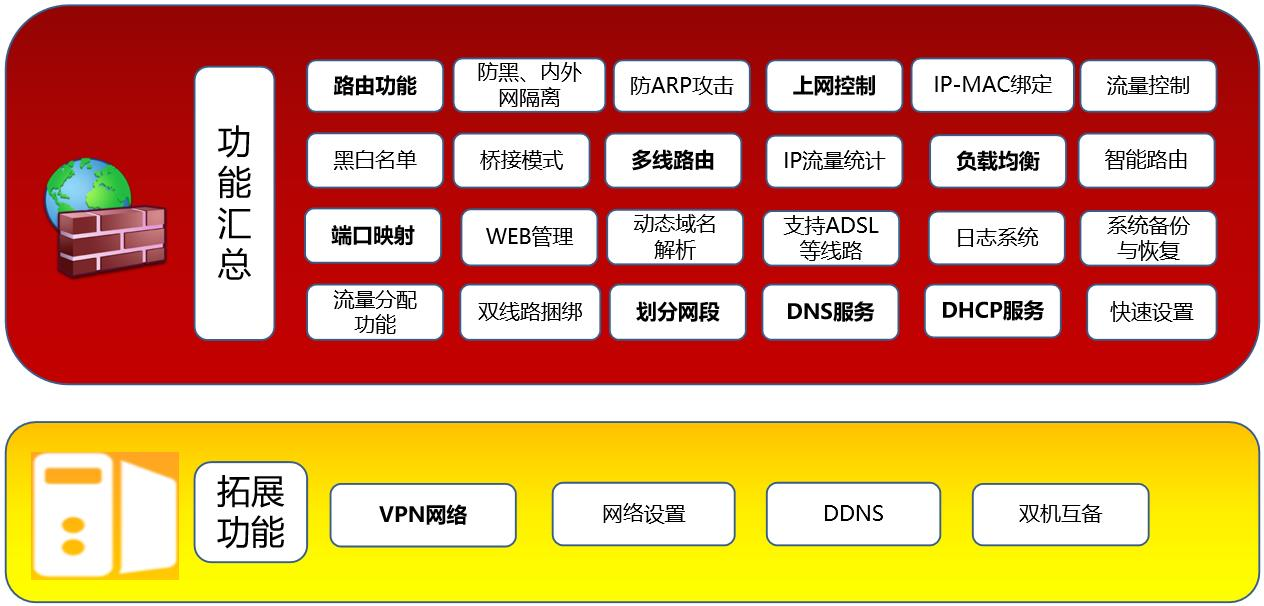﻿ MG系列 邮件+防火墙2合1网关-佑友—邮件系统整体解决方案，邮件服务器，邮件网关，邮件归档

MG系列 邮件+防火墙2合1网关

2019-09-07| 发布者: 河辰佑友 | 查看: |

需求背景

1、企业邮箱不想租空间，但自建又很麻烦，如何才能安全便捷？

2、企业局域网经常出现网络问题，如何解决？

3、怎样建立公司的网络平台，既有便利性，又有安全性和经济型？

邮件+防火墙2合1网关解决方案

佑友邮件+防火墙2合1网关为您提供三大模块组建企业信息化平台：邮件系统模块 、网络安全防火墙、VPN模块

为您提供邮件收发服务，内置多种功能，在帮助您满足日常办公邮件收发需求的同时，也协助您合理有效管理邮件。

费用：企业邮箱一次性购买，终身可用，在公司内部搭建一套高效邮件服务平台，把邮件系统搬到公司内部，由自己管理，信息备份在公司自己服务器上，再也不用担心因忘记续费而造成邮件无法使用，邮件数据放在自己的机房更安全，不再担心信息泄密，让竞争对手得知业务报价，标底等信息的隐患。企业级防火墙+路由，保障您的网络更安全更稳定。弥补传统路由器因内外人数增加带来的网络延迟和不稳定问题；防火墙模块具备了防黑功能，防止ARP等病毒骚扰；佑友邮件+防火墙2合1网关同时还配备了上网行为管理模块，可以合理有效控制员工上网行为，大大降低了员工上网中病毒的概率，同时高效使用公司带宽，不会造成网络阻塞等状况；

对公司内网用户的权限进行定制时，可将用户的地址与计算机的物理地址进行绑定，进一步加强控制的有效性，将每个用户对应于不同的群组，而每个群组又可以自定义相应的权限，这样，就可以对用户上网的权限进行管理了。对内部计算机用户的各种行为进行控制，控制某一用户某一时段是否可以进行QQ、MSN、ICQ、POPO等聊天工具，是否可是在线看电影，是否可以上网，是否可以收发邮件，是否可以上传下载哪些文件，是否可以使用BT等下载工具，是否可以玩在线游戏；只能上哪些网站，不能上哪些网站等等！

外网防御，防病毒攻击

在网络及数据安全性上，佑友邮件+防火墙2合1网关采用业界最先进的状态检测机制，能有效地阻止黑客入侵，对于恶意的网络探测、端口扫描、DOS攻击、DDOS攻击、洪水攻击、死PING、PI伪装等攻击方式，在邮件+防火墙2合1网关上就能直接屏蔽，其底层的安全策略，保障了网络数据的安全。邮件+防火墙2合1网关对流经它的网络通信进行扫描，这样能够过滤掉一些攻击，以免其在目标计算机上被执行。邮件+防火墙2合1网关还可以关闭不使用的端口。而且它还能禁止特定端口的流出通信，封锁特洛伊木马。

VPN功能

VPN功能助您实现异地互联互通，将两地或者多地的网络成为一个局域网，也可以实现外出员工通过VPN网络连接到公司局域网，访问局域网的办公系统。佑友邮件+防火墙2合1网关支持SSL,PPTP等点对网，网对网所有协议；自带传输加密属性，保障您的数据在传输过程中不被泄露。邮件+防火墙2合1网关规格型号说明

 规格 功能特性 线路 普通ADSL(动态IP) Y Y Y Y Y Y Y Y Y ADSL(静态IP) Y Y Y Y Y Y Y Y Y 宽带线路(动态IP) Y Y Y Y Y Y Y Y Y 宽带线路(静态IP) Y Y Y Y Y Y Y Y Y 域名 顶级域名数量 1 1 2 2 3 3 5 20 50 二级域名数量 3 3 3 3 3 3 3 3 3 路由支持 静态路由、策略路由 Y Y Y Y Y Y Y Y Y 基于源地址路由 Y Y Y Y Y Y Y Y Y 接入模式 网关模式 Y Y Y Y Y Y Y Y Y 桥接模式 Y Y Y Y Y Y Y Y Y 旁路模式 Y Y Y Y Y Y Y Y Y 防火墙 共享上网（NAT） Y Y Y Y Y Y Y Y Y 防火墙管理系统 Y Y Y Y Y Y Y Y Y 基于时间段的权限控制 Y Y Y Y Y Y Y Y Y 防黑客攻击 Y Y Y Y Y Y Y Y Y 网址过滤 Y Y Y Y Y Y Y Y Y 日志功能 Y Y Y Y Y Y Y Y Y 端口映射 Y Y Y Y Y Y Y Y Y VPN（点对网,网对网） Y Y Y Y Y Y Y Y Y 流量分配（QOS） Y Y Y Y Y Y Y Y Y 跨三层扫描绑定 Y Y Y Y Y Y Y Y Y ADSL双线路捆绑 Y Y Y Y Y Y Y Y Y SHELL管理系统 Y Y Y Y Y Y Y Y Y 邮箱 支持SMTP、POP3 Y Y Y Y Y Y Y Y Y 支持WEBMAIL Y Y Y Y Y Y Y Y Y 支持WEB邮件管理 Y Y Y Y Y Y Y Y Y 用户数 50 100 200 500 1000 2000 5000 20000 5万 邮件在线杀毒 Y Y Y Y Y Y Y Y Y Web登录界面定制 N N N Y Y Y Y Y Y 邮件域 1 1 2 2 3 3 5 5 5 邮件监控 Y Y Y Y Y Y Y Y Y 垃圾邮件过滤 Y Y Y Y Y Y Y Y Y 全球邮功能 Y Y Y Y Y Y Y Y Y 邮件中继 Y Y Y Y Y Y Y Y Y 防盗号机制 Y Y Y Y Y Y Y Y Y 星标邮件 Y Y Y Y Y Y Y Y Y 大附件 Y Y Y Y Y Y Y Y Y 手机邮件收发 Y Y Y Y Y Y Y Y Y 自动转发 Y Y Y Y Y Y Y Y Y 自动回复 Y Y Y Y Y Y Y Y Y 邮件账户导入导出 Y Y Y Y Y Y Y Y Y 通讯录导入导出 Y Y Y Y Y Y Y Y Y 内、外部邮件域 Y Y Y Y Y Y Y Y Y 邮件收发对象限制 Y Y Y Y Y Y Y Y Y 查询邮件队列 Y Y Y Y Y Y Y Y Y FTP帐号管理功能（含60G空间） Y Y Y Y Y Y Y Y Y 备份 数据库备份 Y Y Y Y Y Y Y Y Y WEB下数据库备份 Y Y Y Y Y Y Y Y Y RAID备份(支持0，1，5方式) N N Y Y Y Y Y Y Y 双机自动备份 N N N N N N N N N 注：Y表示具有此项功能；N表示此功能非标配，如需要则另外报价。型号太多，未能尽录。